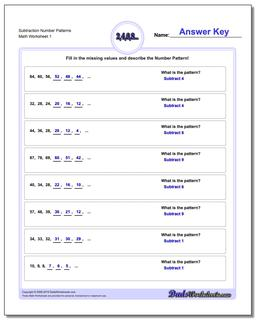# Math Worksheets: Number Patterns: Number Patterns: Subtraction Number Patterns## Subtraction Number Patterns

PropertyValue
DescriptionSubtraction Number Patterns: Number pattern problems using only subtraction operations.
Resource TypeWorksheet